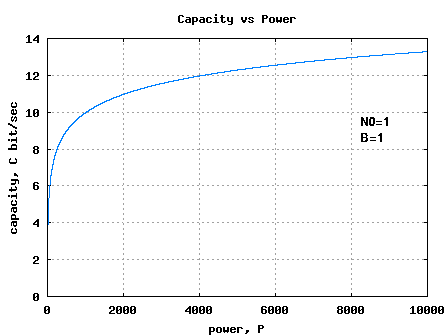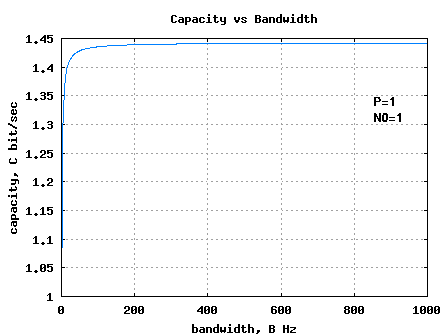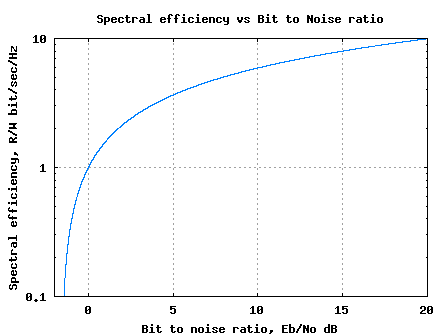- DSP log - http://www.dsplog.com -

Bounds on Communication based on Shannon’s capacity

Posted By Krishna Sankar On June 18, 2008 @ 7:45 am In Channel | 20 Comments

This is the second post in the series aimed at developing a better understanding of Shannon’s capacity equation. In this post let us discuss the bounds on communication given the signal power and bandwidth constraint. Further, the following writeup is based on Section 12.6 from Fundamentals of Communication Systems by John G. Proakis, Masoud SalehiIn the first post in this series, we have discussed Shannon’s equation for capacity of band limited additive white Gaussian noise channel with an average transmit power constraint . The capacity is,

bits/second

where

is the capacity in bits per second, is the bandwidth in Hertz, is the signal power and is the noise spectral density.

## Capacity with increasing signal power

Increasing the signal power will mean that we can split the signal level into more number of levels even while ensuring low probability of error. Hence increasing signal power will lead to more capacity. However, as the increase in capacity is a logarithmic function of power, the returns are diminishing.

 Matlab/Octave script for plotting capacity vs power B=1; N0=1; P= [0:10^4]; C = B.*log2(1+P./(N0*B)); plot(P,C); xlabel('power, P'); ylabel('bandwidth,B'); ylabel('capacity, C bit/sec'); title('Capacity vs Power')Figure: Capacity vs Power, keeping Noise and Bandwidth to unity

Can observe that increase in capacity is diminishing as we keep increase the value of power.

## Capacity with increasing bandwidth

The second variable to play with is the bandwidth. Increasing the bandwidth has two effects:

1. More bandwidth means we can have more transmissions per second, hence higher the capacity.

2. However, more bandwidth also means that there is more noise power at the receiver.

The latter reduces the performance.

Let us try to evaluate the capacity equation when bandwidth tends to infinity i.e
.

From the Taylor series  expansion, we know that

.

Applying this to the above equation,

.

This means that increasing bandwidth alone will not lead to increase of the capacity.
 Matlab/Octave script for plotting capacity vs bandwidth P = 1; N0 = 1; B = [1:10^3]; C = B.*log2(1+P./(N0*B)); plot(B,C) xlabel('bandwidth, B Hz'); ylabel('capacity, C bit/sec'); title('Capacity vs Bandwidth')Figure: Capacity vs Bandwidth, keeping signal power and noise power to unity

Can observe that the maximum achievable capacity by increasing bandwidth is 1.44 times the value.

## Capacity (in bit/sec/Hz) vs Bit to noise ratio (Eb/No)

From our discussion till now, we have understood that a practical communication should have a rate which is lower than capacity , i.e.

bits/second.

Dividing both sides of the equation by bandwidth ,

bits/second/Hz.

Further, from our discussion on Bit error rate for 16PSK modulation using Gray mapping , we know that symbol to noise ratio is times the bit to noise ratio, i.e.
.

Substituting this into the capacity equation,
bits/second/Hz.

For notational convenience, let us define as the spectral efficiency in bits/second/Hertz.

The above equation can be equivalently represented as,

.

In the above equation, when tends to zero, the bit to noise ratio should be,

.

(Thanks to L’Hospital’s rule ).

This means that for reliable communication, we need to have or equivalently expressing in decibels, .

 Matlab/Octave script for plotting the capacity in Bits/sec/Hz vs Bit to noise ratio r = [0:.001:10]; Eb_No_lin = (2.^r -1)./r; Eb_No_dB = 10*log10(Eb_No_lin); semilogy(Eb_No_dB,r) axis([-2 20 0.1 10]); grid on xlabel('Bit to noise ratio, Eb/No dB'); ylabel('Spectral efficiency, R/W bit/sec/Hz') title('Spectral efficiency vs Bit to Noise ratio')Figure: Spectral efficiency vs bit to noise ratio

The above plot captures the equation,

.

It divides the area into two regions:

(a) In the region below the curve, reliable communication is possible and

(b) in the region above the curve, reliable communication is not possible.

Closer the performance of a communication system is to the curve, more optimal is the system.

In the next post in this series, we will discuss the performance of various modulation schemes like BPSK, QPSK, QAM etc by mapping them into various points in the above plot.

## Reference

[COMM-SYS-PROAKIS-SALEHI] Fundamentals of Communication Systems by John G. Proakis, Masoud SalehiURL to article: http://www.dsplog.com/2008/06/18/bounds-on-communication-shannon-capacity/

URLs in this post:

 Fundamentals of Communication Systems by John G. Proakis, Masoud Salehi: http://www.amazon.com/gp/redirect.html?ie=UTF8&location=http%3A%2F%2Fwww.amazon.com%2FFundamentals-Communication-Systems-John-Proakis%2Fdp%2F013147135X&tag=dl04-20&linkCode=ur2&camp=1789&creative=9325

 Shannon’s equation for capacity of band limited additive white Gaussian noise channel with an average transmit power constraint: http://www.dsplog.com/2008/06/15/shannon-gaussian-channel-capacity-equation/

 Taylor series: http://en.wikipedia.org/wiki/Taylor_series#Calculation_of_Taylor_series

 Bit error rate for 16PSK modulation using Gray mapping: http://www.dsplog.com/2008/05/18/bit-error-rate-for-16psk-modulation-using-gray-mapping/

 L’Hospital’s rule: http://en.wikipedia.org/wiki/L

 click here to SUBSCRIBE : http://www.feedburner.com/fb/a/emailverifySubmit?feedId=1348583&loc=en_US